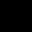# Find the average of the series : 312, 162, 132, 142 and 122?

## One Answer on this Question

1.L.Lalitha priya says:

Sum of all numbers in series= 870 (I.e 312+162+132+142+122)
Average=sum of numbers/count of numbers
Average= 870/5
= 174

Scroll to top